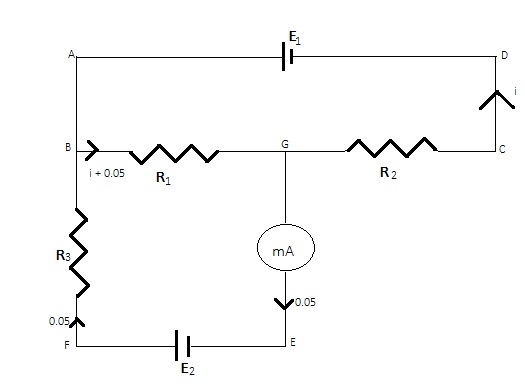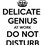# It is Greek to me

The circuit shown in fig. contains three resistors R1 = 100 ohm, R2 = 50 ohm and R3 = 20 ohm and cells of emfs E1 = 2V and E2. The ammeter indicates a current of 50 mA. Determine the currents in the resistors and the emf of the second cell. The internal resistance of the ammeter and that of the cells should be neglected.

Sol| Applying Kirchhoff’s voltage law in the loop of ABCD, we get E1 = (I + 0.05)R1 + IR2 or I = -20 mA Current through R1 is 30 mA toward right. Current through R2 is 20 mA toward left. Applying KVL in loop BGEF, we get E2 = (I + 0.05)100 + (0.05)20 = 8V.. Now my question is, since V = IR, it implies that E1 = IReq.
Or, 2/20mA = Req = 100 ohm, but manually calculating it should be 150 ohm….. where am I mistaken?
Same case is with E2 battery.Note by Swapnil Rajawat
5 years ago

This discussion board is a place to discuss our Daily Challenges and the math and science related to those challenges. Explanations are more than just a solution — they should explain the steps and thinking strategies that you used to obtain the solution. Comments should further the discussion of math and science.

When posting on Brilliant:

• Use the emojis to react to an explanation, whether you're congratulating a job well done , or just really confused .
• Ask specific questions about the challenge or the steps in somebody's explanation. Well-posed questions can add a lot to the discussion, but posting "I don't understand!" doesn't help anyone.
• Try to contribute something new to the discussion, whether it is an extension, generalization or other idea related to the challenge.

MarkdownAppears as
*italics* or _italics_ italics
**bold** or __bold__ bold
- bulleted- list
• bulleted
• list
1. numbered2. list
1. numbered
2. list
Note: you must add a full line of space before and after lists for them to show up correctly
paragraph 1paragraph 2

paragraph 1

paragraph 2

[example link](https://brilliant.org)example link
> This is a quote
This is a quote
    # I indented these lines
# 4 spaces, and now they show
# up as a code block.

print "hello world"
# I indented these lines
# 4 spaces, and now they show
# up as a code block.

print "hello world"
MathAppears as
Remember to wrap math in $$ ... $$ or $ ... $ to ensure proper formatting.
2 \times 3 $2 \times 3$
2^{34} $2^{34}$
a_{i-1} $a_{i-1}$
\frac{2}{3} $\frac{2}{3}$
\sqrt{2} $\sqrt{2}$
\sum_{i=1}^3 $\sum_{i=1}^3$
\sin \theta $\sin \theta$
\boxed{123} $\boxed{123}$

Sort by:

What is the answer that has been given in the textbook?

- 5 years ago

In textbook it is not asked to find the resistance so this answer is also not given.

- 5 years ago